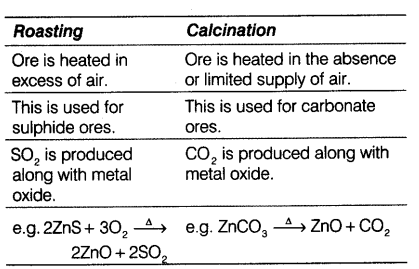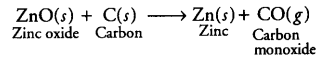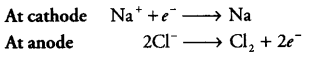# (i) Give differences between roasting and calcination with suitable examples

(i) Give differences between roasting and calcination with suitable examples.
(ii) Explain how the following metals are obtained from their compounds by the reduction process.
(a) Metal M which is in the middle of the reactivity series.
(b) Metal N which is high up in the reactivity series.
Give one example of each type.

1 Like

(i)(ii) (a) The metal M which is in the middle of the reactivity series (such as iron, zinc, lead, copper etc) is moderately reactive. Thus, to obtain such metals from their compounds, their sulphides and carbonates (in which they are present in nature) are first converted into their oxides by the process of roasting and calcination respectively.
The metal oxides (MO) are then reduced to the corresponding metals by using suitable reducing agents such as carbon, e.g. zinc metal can be obtained from its oxide as follows:(b) The metal N which is high up in the reactivity series (such as sodium, magnesium, calcium, aluminium etc) is very reactive and cannot be obtained from its compound by heating with carbon. Therefore, such metals are obtained by electrolytic reduction of their molten salt, e.g. sodium is obtained by the electrolysis of molten sodium chloride (NaCl).Example of each metal is Fe and Na respectively.

1 Like